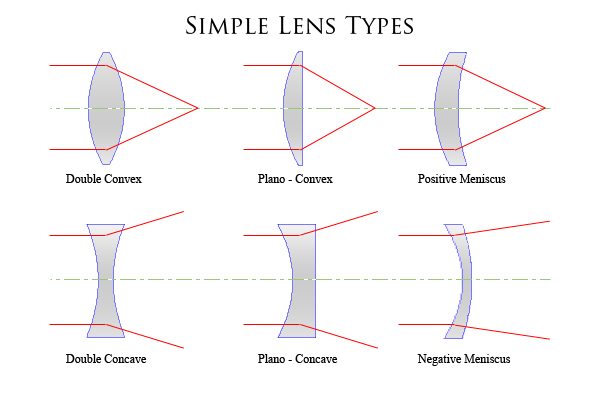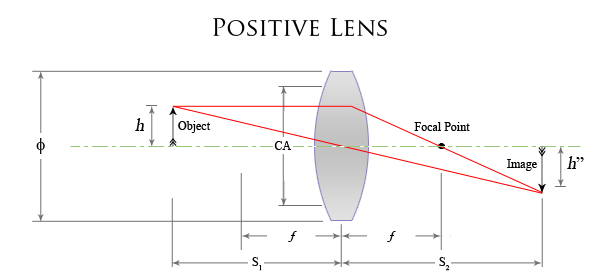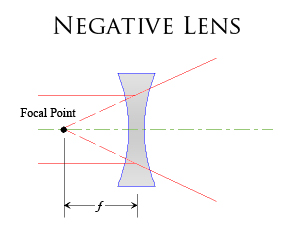Fundamentals of Light & Optics

Lenses & Optics - Lenses

In optics, lenses are an important device allowing us to control and alter the direction of light waves and to focus light to a desired position.

Lenses function by transmitting and refracting light waves in a particular manner determined by the design and shape of the lens itself. The shape of the lens determines how light is refracted, a lens will either converge light rays, i.e. bring to a point or diverge light rays i.e. light rays do not meet.

Lets now take a further detailed look at lenses...

Lens Types

The basic geometry and classification of a lens is by the curvature of the two optical surfaces. Simple lenses are spherical lenses where the two optical surfaces form part of two intersecting spheres.

There are six basic types of lenses as follows;Fig 3.0 - Simple Lenses

Fig 3.0 shows the six basic types of lens geometry. Lenses are further classified into two sub-groups, Negative lenses and Positive lenses.

Positive and negative refers to the focal point of a lens. A negative lens has a focal length with a negative value.

Positive Lenses

Positive lenses typically have a center cross-section which is greater in thickness than that at the edge. The combined effects of refraction at the front and back surfaces of the lens will bend light to a point or Focus - The light from this type of lens is focused to a point in front of the lens.

Positive lenses produce a real image, - the image produced by this type of lens can be projected onto a screen.

The three basic positive lenses are:

• Double Convex lens
• Plano - Convex lens
• Positive Meniscus lensFig 3.1 - Positive Lens

Negative Lenses

Negative lenses are lenses which are thinner at the centre than the edge. The same optic laws apply to negative lenses as they do with positive lenses, although a negative lens works in a somewhat different way than a positive lens.

The focal lengths of this type of lens are negative, this can be worked out mathematically by assigning a negative radii of curvature to a concave lens surface. If you pass parallel light rays through a negative lens, they seem to spread out from a point behind the lens, the distance from this point to the centre line of the lens is the focal length; this is given a negative value, as it is on the opposite side of the lens from the focal point of a positive lens.

Because negative lenses do not bring parallel light rays together, they DO NOT produce a real image, but they do produce a virtual image.

The three basic negative lenses are:

• Double Concave lens
• Plano - Concave lens
• Negative Meniscus lensFig 3.2 - Negative Lens

Fig 3.2 demonstrates some of the important aspects of a negative lens. A negative lens produces a virtual image, and the focal point is behind the lens.

Fig 3.2 demonstrates some of the important aspects of a negative lens. A negative lens produces a virtual image, and the focal point is behind the lens.

In the next section, we will investigate the basic lenses which form parts of more complex optical systems..

This Document Complies to W3C XHTML 1.0 Strict Standards